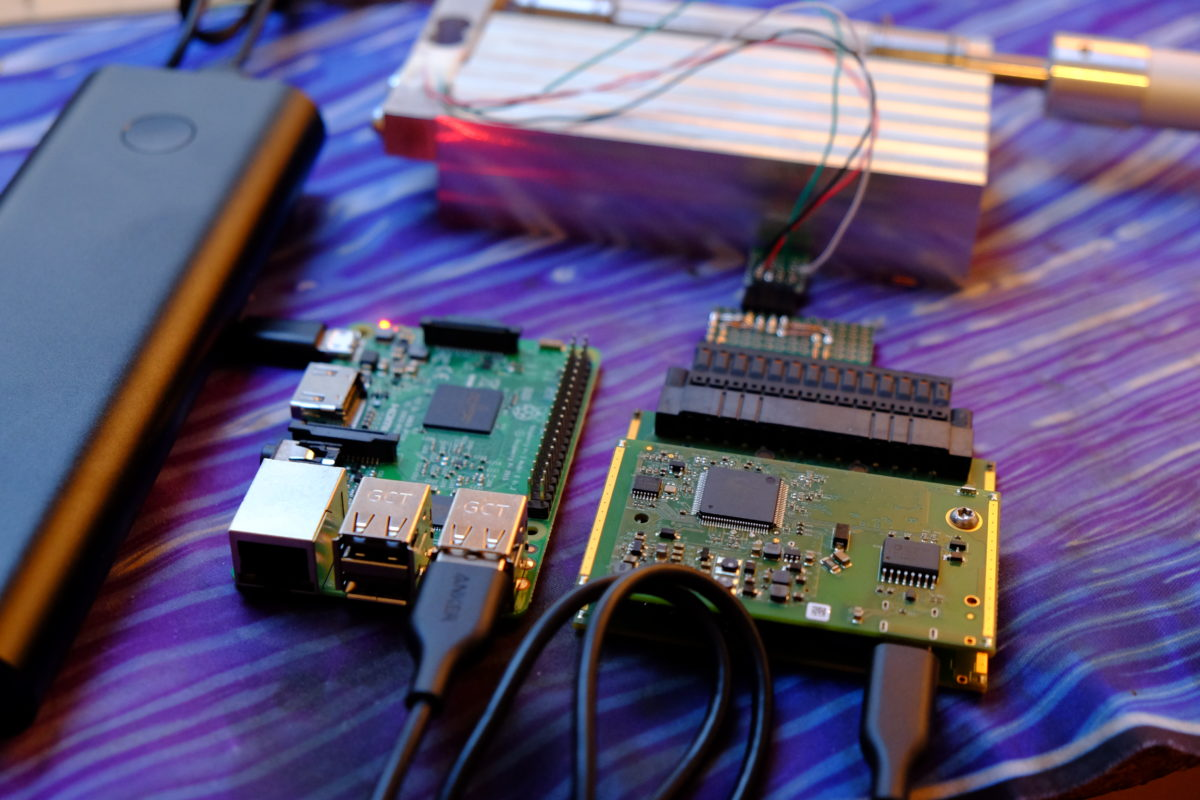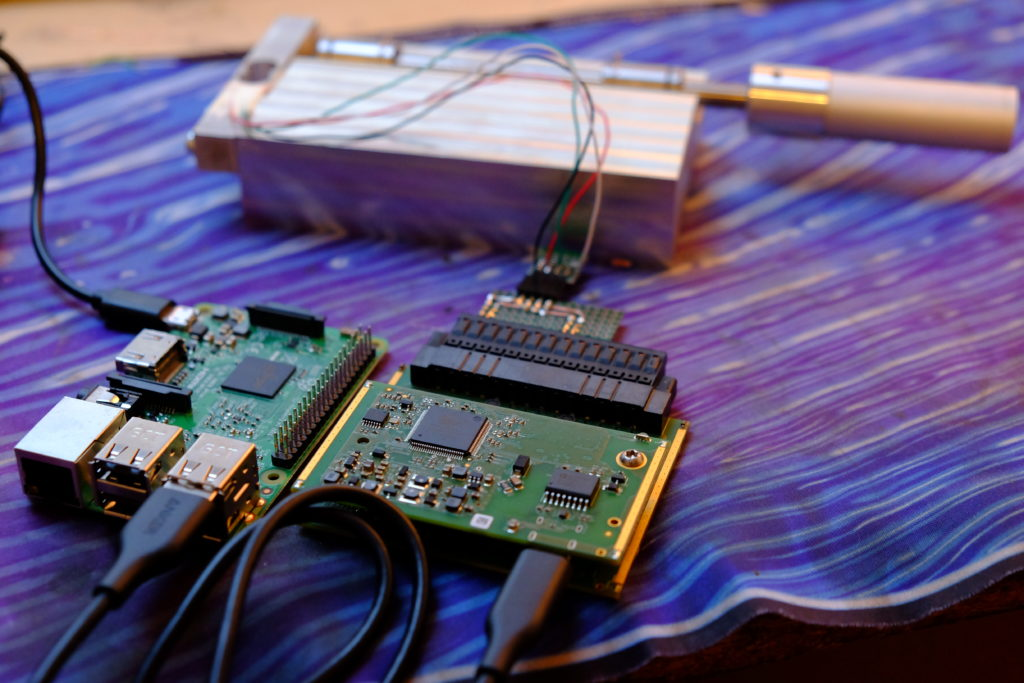# Processing MonoDAQ-U-X data in python on Raspberry Pi

#### Python is one of the most popular programming languages and for good reason, it is incredibly versatile and a very powerful tool for data processing. Let’s take a look at how to use python running on a Raspberry Pi to receive and process data from the MonoDAQ U-X.

Before starting with python make sure you have completed the first part of this tutorial. IDM software must have been installed and the U-X detected by it. While the tutorial focuses on python running on the Raspberry Pi most information here can also be applied to python running on other machines.

### Python

#### Before we start writing code

Before we can start writing python code we need to install a few plugins. The insallation is straight forward and can be accomplished with a single command. Open up the terminal and run:

``python3 -m pip install jupyter bokeh isotel-idm ``

Make sure that you are installing the plugins on python 3.x since some won’t work with python 2.x

It will take a couple of minutes to complete since we are installing quite a few modules but eventually you should get a message that everything has been installed.

After the modules have been set up run the IDM software and make sure the U-X has been detected properly. Follow this manual to set up the IDM software if you don’t have it set up already.

#### Starting to code

We will be using the IDLE development enviroment since it comes preinstalled with Raspbian. Start IDLE and open a new document.

First, we will import the required modules which we have downloaded previously.

``from isotel.idm import monodaq, signal, gateway``

To makes things easier we shorten monodaq.MonoDAQ_U() to mdu since we will use it often in our code.

``mdu = monodaq.MonoDAQ_U() ``

It is advisable to use the reset command to start a fresh channel setup.

``mdu.reset() ``

#### Configuring channels

To configure the channels use the following notation:

`` mdu['parameter we want to change'] = 'desired value'``

Just like in the first part of this guide series we will be measuring temperature with a thermocouple. We want to configure the first channel to be a thermocouple input so the command looks like:

``mdu['ch.function.function0'] = 'Thermocouple'``

Keep in mind that pins on the U-X start with 1 but counting in IDM starts at 0 so to set the function of the first U-X pin we need to set the value of function0 and not function1!

The easiest way to figure out what exactly should go in the square brackets is having the parameter tree open and following the structure.

For example, let’s say we wanted to set channel 3 to be a PWM output with a 1000Hz frequency. We have already shown how to change the function of a channel. To figure out how to change the rate we need to take a look at where rate2 is located in the parameters tree.

Following the structure of the tree (ignoring the first var folder) we can see that the command to change the rate will be:

``mdu['ch.rate.rate2'] = 1000``

The same logic applies for changing all other chanel parameters.

Before starting measuring we need to make sure the U-X has self-calibrated so we use:

``mdu.wait4ready()``

We can check if everything has been set up as we wanted by printing the setup with:

``mdu.print_setup()``

#### Obtaining the measured values

##### Fetch data

To obtain the measured values from the channels we use the mdu.fetch(‘Number of samples’) command. For example, to collect 100 samples:

``mdu.fetch(100)``

The fetch command will wait until the desired number of samples have been taken and then return them all at the same time.

##### stream2signal

To convert the data into a convenient form we use the signal2stream command. This command creates a dictionary from the fetched data and also can be used for real time logging which we will explore later.

``signal.stream2signal(mdu.fetch(100))``

In reality, we are greatly simplifying things. Fetch and stream2signal methods are actually generators. Also, the fetch command provides us with additional information besides just the measured values like voltage or temperature.

In order to keep this guide short and easy to follow will spare you the gory details. But if you want to know what’s really going on here head over to Isotel’s page which contains more detailed information.

For now all you need to know is that a simple way to obtain a dictionary containing measured data is by using:

``````for data in signal.stream2signal(mdu.fetch(10)):
allofyourneatelystoreddata = data``````

This might seem a bit confusing if you are used to for loops well… looping. But as a consequence of working with generators, the above code does not actually loop. It just runs once and is not constantly overwriting allofyourneatlystoreddata as you might have expected.

If we print allofyourneatelystoreddata you can see that it is now stored as a dictionary. In fact, it is a nested dictionary!

##### Dictionary to list

To retreive the data from the dictionary we need to provide it with the dictionary keys which are channel name and axis that we are interested in.

``````for data in signal.stream2signal(mdu.fetch(100)):
print(data['Channel']['Axis'])``````

You can figure out what exactly to insert in place of ‘Channel’ by looking at the channel setup in IDM and combining the pin and unit into a single string. The unit should be put in to square brackets and seperated from pin name by a single space.

Alternatively, just print the entire dict (print(data)) and read what the keys are. ‘Axis’ should just be ‘y’ for whatever you are measuring (e.g. temperature) or ‘x’ for time of measurement.

For example, in order to obtain (or in this case print) a list of temperatures, we would use:

``````for data in signal.stream2signal(mdu.fetch(10)):
print(data['TC1+ [oC]']['y'])``````

#### Writing a simple program

So let’s put all of what we learned together and write a simple program which will record a thermocouple input for 5 seconds at 100Hz. Afterward, it will extract the necessary data to draw a pyplot of temperature with respect to time.

``````from isotel.idm import monodaq, signal, gateway
import matplotlib.pyplot as plt

mdu = monodaq.MonoDAQ_U()
mdu.reset()  # Resets any previous channel configurations
mdu['ch.function.function0'] = 'Thermocouple'  # Sets channel function
mdu['ch.rate.rate0'] = 100  # Sets sample rate
mdu.wait4ready()  # Waits for the U-X to calibrate
mdu.print_setup()  # Prints the new channel setup

print('Starting Measurement')  # Start of measurement
for data in signal.stream2signal(mdu.fetch(500)):
temperature_list = data['TC1+ [oC]']['y']  # Saves temperature data as a list
time_list = data['TC1+ [oC]']['x']  # Saves time data as a list
print('Measurement Complete')  # End of measurement

plt.plot(time_list, temperature_list)  # Plots the graph
plt.ylabel('Temperature [oC]')  # Label the axis
plt.xlabel('Time [s]')
plt.show()  # Show the graph``````

When the program notifies us that the measurement has begun we heated up the thermocouple with a lighter. This is the result:

In this example we just plotted the data but once you have converted it in to a dictionary the possibilities are endless. You can even export it as a .csv import it in to X3 on another machine and do further analysis there.

#### Acquiring data in real-time

Having to provide how many samples should be taken in advance is often not very practical. Also, being able to see the measured values in real-time would be handy. So let’s take a look at how we can accomplish both.

Remember the stream2signal method?

Well by adding a split parameter to the stream2signal we can make it send out smaller chunks of data which we can use for real-time monitoring. We make sure that fetch won’t run out of data by giving it a very large number of samples to acquire. For example:

``````for some_data in signal.stream2signal(mdu.fetch(1000000), split=100):
do something``````

Unlike in the previous example, this time the for loop acts like we would expect it to. Assuming the sample rate is set at 100Hz the code in the loop will run once per second with some_data being a dictionary containing the last 100 samples.

We could add some_data to a list containing all of the measurements for storage or later analysis. But we could also use some_data to display current values of measured variables or even plot it in real-time.

Since fetch has been given a high value the measurement will be going on for a long time. In order to stop it we use the atexit module

``````import atexit
def goodbye():
print("saving. goodbye")
atexit.register(goodbye)``````

Atexit allows us to stop the measurement at any time by pressing ctrl+c. After ctrl+c is pressed code in goodbye() runs and the program closes.

#### Real-time example program

Below is an example program you can test out. Like the first program, the channels have been set up to measure a thermocouple input at 100Hz. But unlike before the user can see the current temperature which is being updated once per second and they can stop the measurement at any time by pressing ctrl+c. When the user stops the measurement the plots are drawn.

``````from isotel.idm import monodaq, signal, gateway  # Import modules
import matplotlib.pyplot as plt
import numpy as np
import atexit

def goodbye():  # Code that runs before program closes (when ctrl+c is pressed)
print('Measurement Complete')
plt.plot(time_list, temperature_list)
plt.ylabel('Temperature [oC]')
plt.xlabel('Time [s]')
plt.show()
atexit.register(goodbye)

temperature_list = []  # Lists for storing all samples
time_list = []
mdu = monodaq.MonoDAQ_U()
mdu.reset()
mdu['ch.function.function0'] = 'Thermocouple'
mdu['ch.rate.rate0'] = 100
mdu.print_setup()

print('Starting Measurement')
for some_data in signal.stream2signal(mdu.fetch(1000000), split=100):
temperature_list += some_data['TC1+ [oC]']['y']
time_list += some_data['TC1+ [oC]']['x']
print("Temperature: ",
np.average(some_data['TC1+ [oC]']['y']),  # Calculate current temperature
'deg C')
``````

### Example of real life application

To give you some inspiration here is a use case where this sort of setup comes in very handy.

In amateur rocketry, a high-performance rocket motor is the most important part of the rocket. The key performance parameters being thrust, total impulse and motor efficiency. Advanced ‘hobbyists’ make their own rocket motors but often don’t have a good way of measuring the motor’s performance.

This is where the U-X and RPi combination comes in. Generally speaking, standing next to a burning rocket motor is not a terrific idea. The operator would ideally like to get some distance between them and the potentially explosive rocket motor.

So here is the set up:

The U-X and the RPi are connected just like we discussed in this guide. A simple thrust measuring rig based on a load cell is constructed and connected to the U-X. Everything is powered with a USB power bank making the system very portable.The U-X removed from its enclosure and connected to the Raspberry Pi before being mounted in a case.

Thrust force is measured with the U-X and being sent over to RPi. RPi is running python code which is storing the data as a .csv file. The RPi and the operator’s laptop are connected to a hotspot. Data logging is initiated from the laptop. After the motor test, the .csv is sent over the network to the laptop where it can be analyzed using Dewesoft’s X3 software.

From the trust data, the total impulse can be calculated. Weighing the motor before and after the test allows the motor efficiency to be calculated providing all of the necessary information. The sample rate is even high enough that any combustion instability will show up in the data which is of great help when optimizing the motor design.

All od this allows the operator and their PC to be a safe distance away from the motor when the test is runni while still obtaining all of the neceserr data. The U-X and RPi combination is even small enough to be mounted in an actual rocket which could enable in flight measurements.

### Conclusion

We have covered all the basics you need to know to get data flying from the U-X to your python program. This opens up infinite possibilities in what you can do and gives you tremendous power. Use it wisely!